Create a new printableMath Worksheets

Sample - Click above to make a new math worksheet (PDF).
 Name _____________________________Date ___________________
 1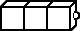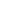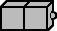3  +  2  =  _____
 2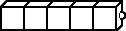2  +  5  =  _____
 3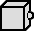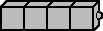1  +  4  =  _____
 4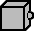5  +  1  =  _____

 1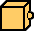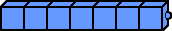_____  +  _____  =  _____
 2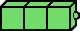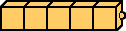_____  +  _____  =  _____
 3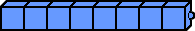_____  +  _____  =  _____
 4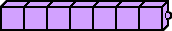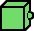_____  +  _____  =  _____

Fill in the missing numbers to complete the addition fact.
1.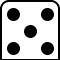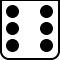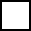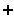2.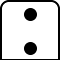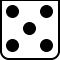3.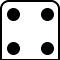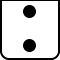Key #2
 aKey #2
Write the sum.
1.
 4 + 1
2.
 5 + 3
3.
 0 + 5
4.
 1 + 0
5.
 2 + 4
6.
 3 + 2
7.
 2 + 1
8.
 1 + 2
9.
 4 + 5
10.
 0 + 4

Write the sum.
 1 4  +  5  =  ____
 2 0  +  0  =  ____
 3 1  +  4  =  ____
 4 5  +  2  =  ____
 5 2  +  3  =  ____
 6 3  +  1  =  ____
 7 0  +  1  =  ____
 8 4  +  2  =  ____
 9 5  +  0  =  ____

Fill in the missing numbers to complete the addition fact.
1.
 0 +5
2.+ 2 6
3.
 1 +2
4.+ 4 9
5.+ 0 3

 Key #2
 aKey #2
Write the sum.
1.
 4 + 5
2.
 5 + 3
3.
 7 + 3
4.
 6 + 3
5.
 3 + 4
6.
 5 + 5
7.
 3 + 3
8.
 4 + 6
9.
 6 + 4
10.
 3 + 5

Write the sum.
 1 5  +  5  =  ____
 2 7  +  3  =  ____
 3 3  +  7  =  ____
 4 6  +  3  =  ____
 5 4  +  6  =  ____
 6 5  +  3  =  ____
 7 3  +  5  =  ____
 8 6  +  4  =  ____
 9 4  +  4  =  ____

Fill in the missing numbers to complete the addition fact.
1.
 6 +10
2.
 3 +8
3.+ 3 8
4.+ 3 10
5.+ 5 9

 Key #2
 aKey #2
Write an addition sentence to solve each word problem.
1.
 The small classroom holds 4 computers on one side and 4 more on the other side. How many computers are there in all? _____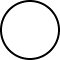_______________ computers
2.
 There are 2 boys and 5 girls. How many kids are there? ____________________ kids

Write the sum.
 1 3  +  7  =  ____
 2 4  +  2  =  ____
 3 5  +  5  =  ____
 4 8  +  1  =  ____
 5 9  +  1  =  ____
 6 1  +  3  =  ____
 7 6  +  2  =  ____
 8 7  +  3  =  ____
 9 2  +  6  =  ____

Write an addition sentence to solve each word problem.
1.
 Dylan has 2 board games and 5 video games. How many games does Dylan have? ____________________ games
2.
 There were 6 fishing boats in the lake and 4 speed boats. How many boats were there in all? ____________________ boats